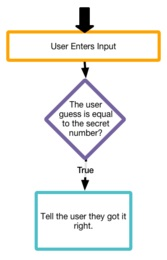## if 如果判断语句

`if (condition) { 执行代码 }`

`if 如果判断语句` 语句需要你指定一个可以返回布尔类型的表达式，可以返回 true 或 false。在这个表达式里，你可以使用 比较操作符（ `<``<=``==``>``>=``!=` ） 或者 逻辑操作符（`&&``||``!` ）。

`if 如果判断语句` 语句通常将条件成立时要执行的代码放在 `{}`。如果满足条件，`{}` 里的代码会从上往下依次执行一遍。

### 示例代码

``````
// 弹窗输入一周工作时间，判断是否超时
var hoursWorked = promptNum("How many hours did you work this week?");
if (hoursWorked > 40) {
write("You worked " + (hoursWorked-40) + " hours overtime.");
}
``````

``````
// 生产随机数字，并让用户在弹窗中猜一下，看是否猜中
var secretNumber=randomNumber(1,10);
var guess = promptNum("Guess my number from 1 to 10.");
if (secretNumber == guess) {
write("You guessed it!");
}
write("Thanks for playing.");
````````````
// 检查是否有 5 次 点击，如有删除按钮控件
var count = 0;
button("id", "Click Me!");
onEvent("id", "click", function(event) {
count = count + 1;
if (count == 5) {
deleteElement("id");
write("Five clicks and you are done.");
}
});
``````

### 语法规则

``````
if (condition) {
statement
}
``````

### 参数说明

condition boolean 表达式 Yes 返回 boolean 布尔值 true 或 false 的表达式。比较操作符包含 `<``<=``==``>``>=``!=` 。boolean 布尔操作符 包含 `&&` `||` `!`
statement Applab 执行语句 Yes Applab 里的执行语句

### 提示说明

• 跟事件捕捉处理不一样，APP 程序并不会对 `if 如果判断语句` 里的条件时刻进行监控。`if 如果判断语句` 代码跟别的普通代码一样，代码执行到这里才进行处理。代码不执行到这里就不会被触发。
• `=` 是赋值操作符。`==` 是判断两个值是否相等的比较操作符。不要混淆哦！

• UI Controls
• Canvas
• Data
• Turtle
• Control
• Math
• Variables
• Functions